# Testing Mutable Objects CS 5010 Program Design Paradigms

• Slides: 18Key Points for Lesson 10. 7 • State makes testing harder. • To test a stateful system, create scenarios that create objects, send them sequences of messages, and then check the observable outputs. • Good OO designs use as little state as possible. 2State makes testing harder • You have to get things into the state you want • Then observe the relevant portions of the final state (at just the right time!) • May want to test a sequence of states • In real world, may have to do tear-down to prepare for next test. – Living in a mostly-functional world makes this unnecessary for us. 3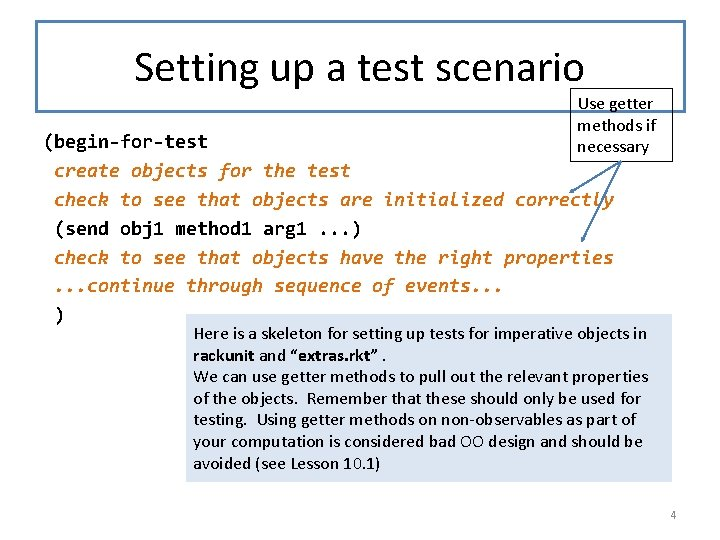Setting up a test scenario Use getter methods if necessary (begin-for-test create objects for the test check to see that objects are initialized correctly (send obj 1 method 1 arg 1. . . ) check to see that objects have the right properties. . . continue through sequence of events. . . ) Here is a skeleton for setting up tests for imperative objects in rackunit and “extras. rkt”. We can use getter methods to pull out the relevant properties of the objects. Remember that these should only be used for testing. Using getter methods on non-observables as part of your computation is considered bad OO design and should be avoided (see Lesson 10. 1) 4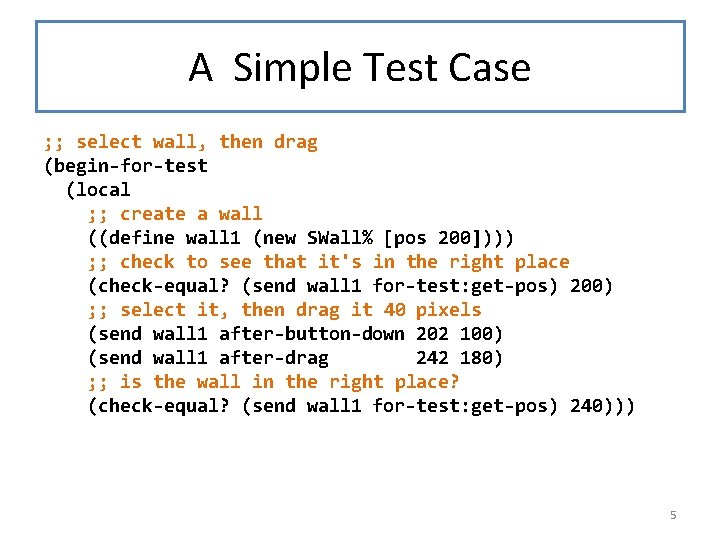A Simple Test Case ; ; select wall, then drag (begin-for-test (local ; ; create a wall ((define wall 1 (new SWall% [pos 200]))) ; ; check to see that it's in the right place (check-equal? (send wall 1 for-test: get-pos) 200) ; ; select it, then drag it 40 pixels (send wall 1 after-button-down 202 100) (send wall 1 after-drag 242 180) ; ; is the wall in the right place? (check-equal? (send wall 1 for-test: get-pos) 240))) 5Another simple test case ; ; don't select wall, then try to drag it (begin-for-test (local ; ; create a wall ((define wall 1 (new SWall% [pos 200]))) ; ; check to see that it's in the right place (check-equal? (send wall 1 for-test: get-pos) 200) ; ; button-down, but not close enough (send wall 1 after-button-down 208 100) (send wall 1 after-drag 242 180) ; ; wall shouldn't move (check-equal? (send wall 1 for-test: get-pos) 200))) 6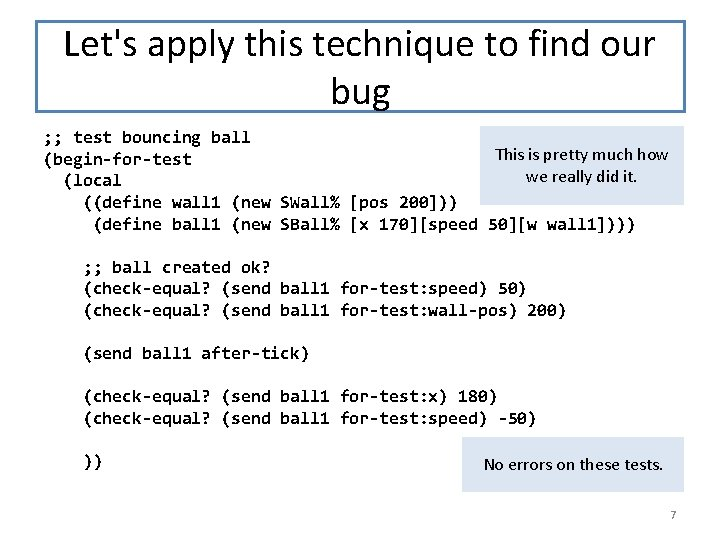Let's apply this technique to find our bug ; ; test bouncing ball This is pretty much how (begin-for-test we really did it. (local ((define wall 1 (new SWall% [pos 200])) (define ball 1 (new SBall% [x 170][speed 50][w wall 1]))) ; ; ball created ok? (check-equal? (send ball 1 for-test: speed) 50) (check-equal? (send ball 1 for-test: wall-pos) 200) (send ball 1 after-tick) (check-equal? (send ball 1 for-test: x) 180) (check-equal? (send ball 1 for-test: speed) -50) )) No errors on these tests. 7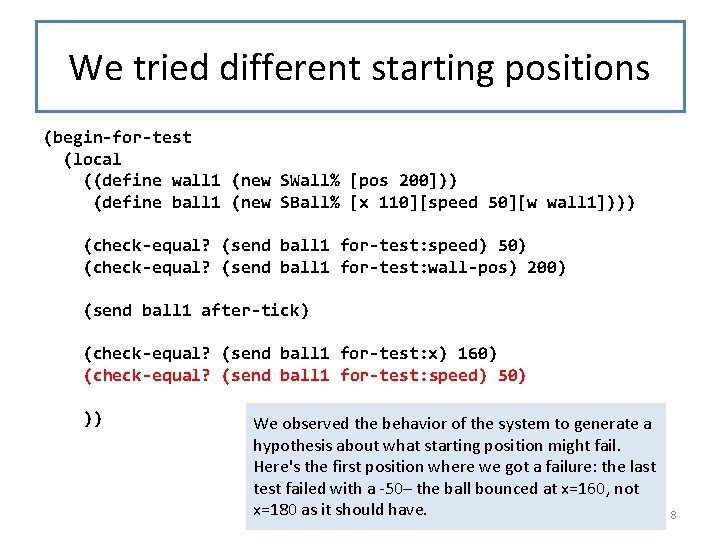We tried different starting positions (begin-for-test (local ((define wall 1 (new SWall% [pos 200])) (define ball 1 (new SBall% [x 110][speed 50][w wall 1]))) (check-equal? (send ball 1 for-test: speed) 50) (check-equal? (send ball 1 for-test: wall-pos) 200) (send ball 1 after-tick) (check-equal? (send ball 1 for-test: x) 160) (check-equal? (send ball 1 for-test: speed) 50) )) We observed the behavior of the system to generate a hypothesis about what starting position might fail. Here's the first position where we got a failure: the last test failed with a -50– the ball bounced at x=160, not x=180 as it should have. 8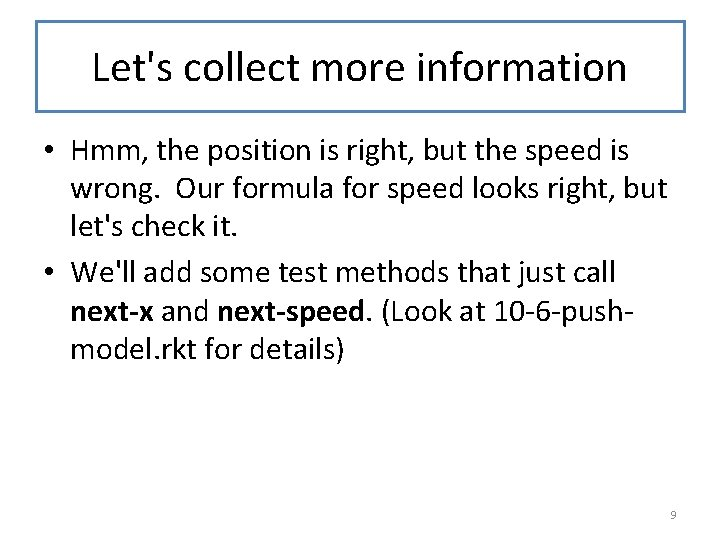Let's collect more information • Hmm, the position is right, but the speed is wrong. Our formula for speed looks right, but let's check it. • We'll add some test methods that just call next-x and next-speed. (Look at 10 -6 -pushmodel. rkt for details) 9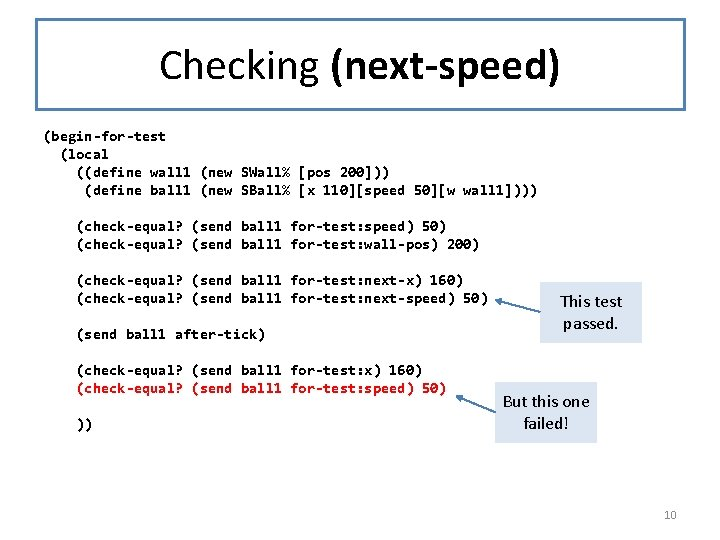Checking (next-speed) (begin-for-test (local ((define wall 1 (new SWall% [pos 200])) (define ball 1 (new SBall% [x 110][speed 50][w wall 1]))) (check-equal? (send ball 1 for-test: speed) 50) (check-equal? (send ball 1 for-test: wall-pos) 200) (check-equal? (send ball 1 for-test: next-x) 160) (check-equal? (send ball 1 for-test: next-speed) 50) (send ball 1 after-tick) (check-equal? (send ball 1 for-test: x) 160) (check-equal? (send ball 1 for-test: speed) 50) )) This test passed. But this one failed! 10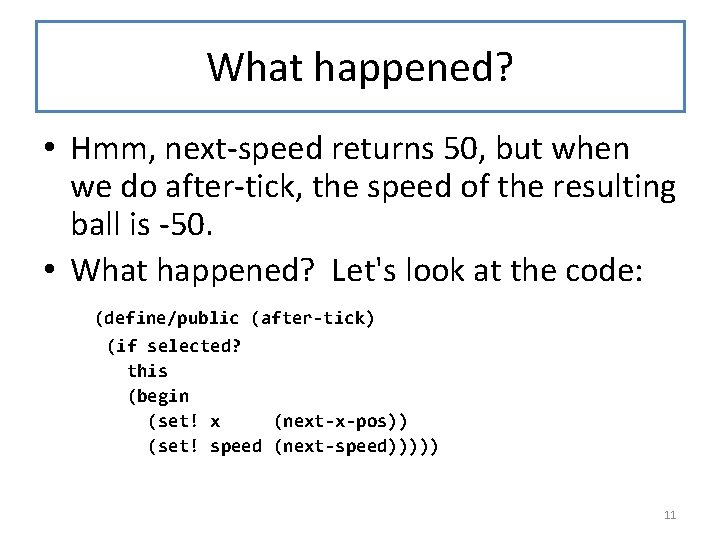What happened? • Hmm, next-speed returns 50, but when we do after-tick, the speed of the resulting ball is -50. • What happened? Let's look at the code: (define/public (after-tick) (if selected? this (begin (set! x (next-x-pos)) (set! speed (next-speed))))) 11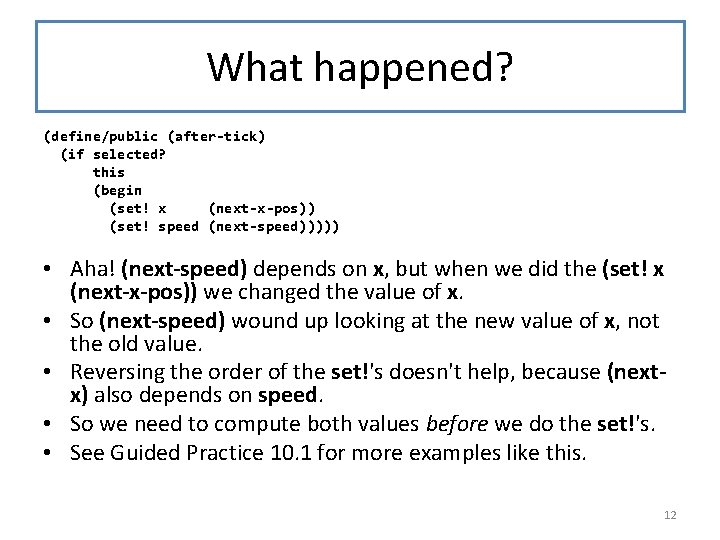What happened? (define/public (after-tick) (if selected? this (begin (set! x (next-x-pos)) (set! speed (next-speed))))) • Aha! (next-speed) depends on x, but when we did the (set! x (next-x-pos)) we changed the value of x. • So (next-speed) wound up looking at the new value of x, not the old value. • Reversing the order of the set!'s doesn't help, because (nextx) also depends on speed. • So we need to compute both values before we do the set!'s. • See Guided Practice 10. 1 for more examples like this. 12Here's the fixed code (define/public (after-tick) (if selected? this (let ((x 1 (next-x-pos)) (speed 1 (next-speed))) (begin (set! speed 1) (set! x x 1))))) (next-x-pos) and (nextspeed) both need the old values of x and speed, so we compute them both and THEN change the values of x and speed. See 10 -7 -push-model-fixed. rkt 13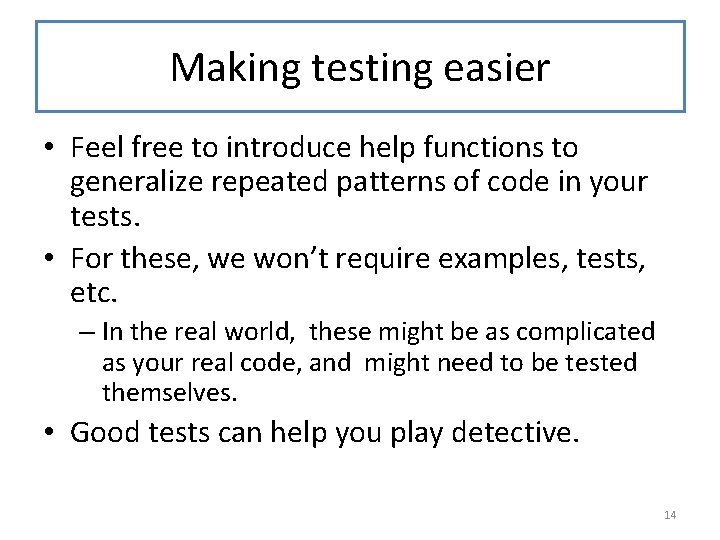Making testing easier • Feel free to introduce help functions to generalize repeated patterns of code in your tests. • For these, we won’t require examples, tests, etc. – In the real world, these might be as complicated as your real code, and might need to be tested themselves. • Good tests can help you play detective. 14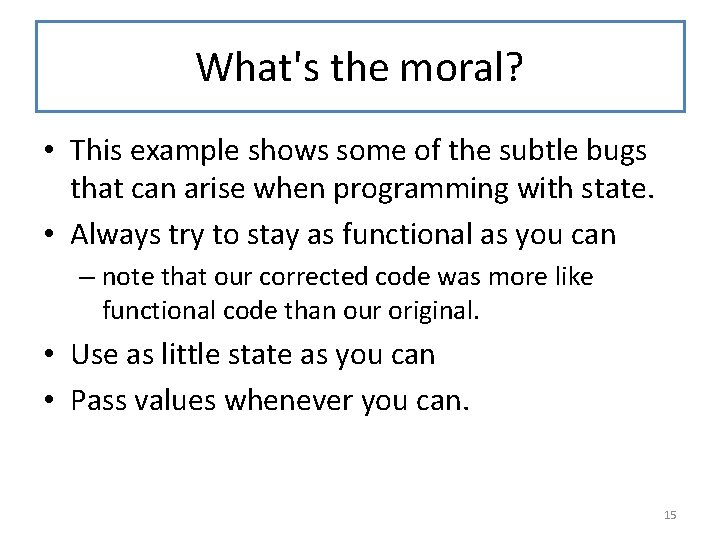What's the moral? • This example shows some of the subtle bugs that can arise when programming with state. • Always try to stay as functional as you can – note that our corrected code was more like functional code than our original. • Use as little state as you can • Pass values whenever you can. 15Java Guru on State: Keep the state space of each object as simple as possible. If an object is immutable, it can be in only one state, and you win big. You never have to worry about what state the object is in, and you can share it freely, with no need for synchronization. If you can't make an object immutable, at least minimize the amount of mutation that is possible. This makes it easier to use the object correctly. As an extreme example of what not to do, consider the case of java. util. Calendar. Very few people understand its statespace -- I certainly don't -- and it's been a constant source of bugs for years. -- Joshua Bloch, Chief Java Architect, Google; author, Effective Java Here’s a quotation on state from a famous Java programmer. 16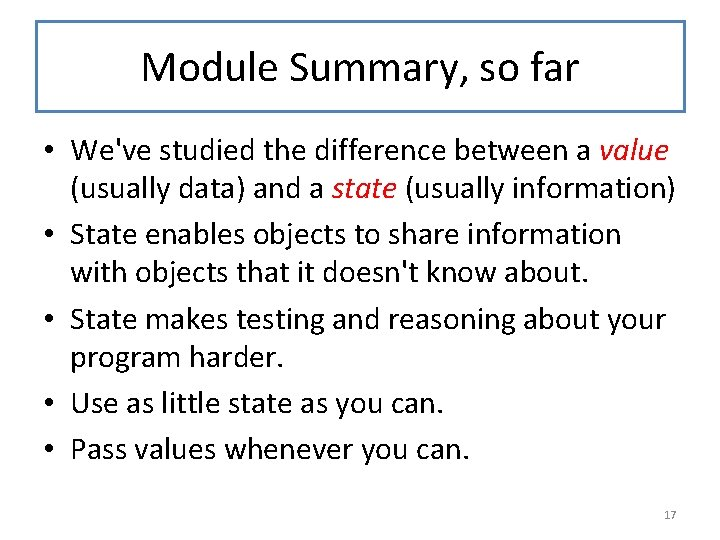Module Summary, so far • We've studied the difference between a value (usually data) and a state (usually information) • State enables objects to share information with objects that it doesn't know about. • State makes testing and reasoning about your program harder. • Use as little state as you can. • Pass values whenever you can. 17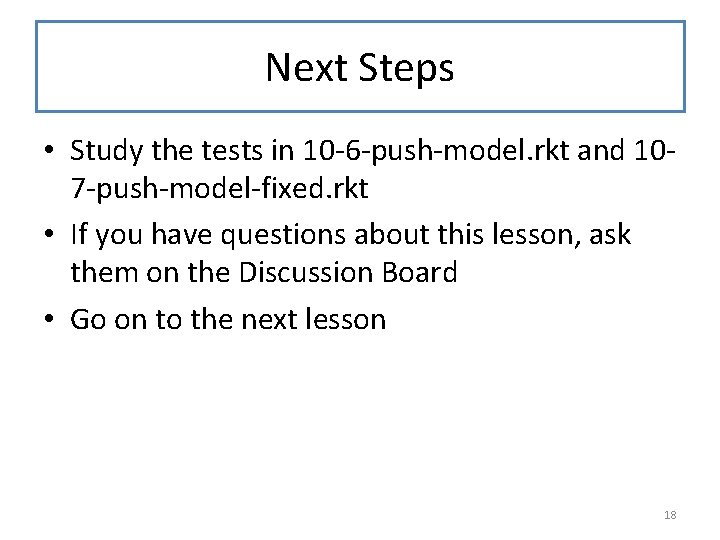Next Steps • Study the tests in 10 -6 -push-model. rkt and 107 -push-model-fixed. rkt • If you have questions about this lesson, ask them on the Discussion Board • Go on to the next lesson 18